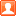##用戶名 Email 自動登錄 找回密碼 密碼 註冊

# 酷！圖片龍捲風特效，看你的網頁在跳舞?brian 發表於 2013-11-16 16:40 | 顯示全部樓層 |閱讀模式
 沒看過吧，網頁也可以颳龍捲風喔！其實下面這個只是一段JavaScript網頁特效，只要我們把他貼到IE瀏覽器的網址列，透過這些參數來改變原本網頁中的圖片位置，產生一個讓人驚艷的圖片翻轉特效，雖然實際用途不大，不過滿有趣的。 第1步 首先，開啟你要展示的網頁，然後將下面這段程式碼，完整的複製並貼上在IE瀏覽器的「網址」列。 javascript:R=0; x1=.1; y1=.05; x2=.25; y2=.24; x3=1.6; y3=.24; x4=300; y4=200; x5=300; y5=200; DI=document.images; DIL=DI.length; function A(){for(i=0; i-DIL; i++){DIS=DI[ i ].style; DIS.position='absolute'; DIS.left=Math.sin(R*x1+i*x2+x3)*x4+x5; DIS.top=Math.cos(R*y1+i*y2+y3)*y4+y5}R++}setInterval('A()',5); void(0);複製代碼第2步 貼上後，直接按下〔ENTER〕，讓他開始執行。第3步 如圖，原本網頁上的一堆圖片開始颳起龍捲風囉！很特別吧。看完，覺得無聊，想停止的話，請直接關掉網頁即可。您需要登錄後才可以回帖 登錄 | 註冊 本版積分規則 回帖後跳轉到最後一頁

GMT+8, 2020-9-24 04:13